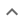•# Tiger Algebra Calculator

We think you wrote:

# No solutions found### Try this:

We are constantly updating the types of the problems Tiger can solve, so the solutions you are looking for could be coming soon!

## Step by Step Solution### Rearrange:

Rearrange the equation by subtracting what is to the right of the equal sign from both sides of the equation :

f*(x)-(2*x+3)=0

## Step  1  :

#### Solving a Single Variable Equation :

Solve   fx-2x-3  = 0

In this type of equations, having more than one variable (unknown), you have to specify for which variable you want the equation solved.

We shall not handle this type of equations at this time.